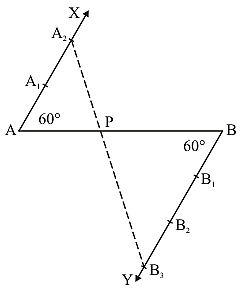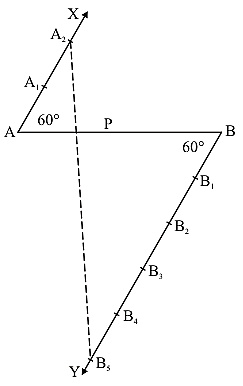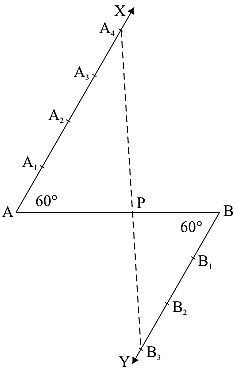×#### Thank you for registering.

One of our academic counsellors will contact you within 1 working day.

Click to Chat

1800-1023-196

+91-120-4616500

CART 0

• 0

MY CART (5)

Use Coupon: CART20 and get 20% off on all online Study Material

ITEM
DETAILS
MRP
DISCOUNT
FINAL PRICE
Total Price: Rs.

There are no items in this cart.
Continue Shopping• Complete JEE Main/Advanced Course and Test Series
• OFFERED PRICE: Rs. 15,900
• View Details

```Chapter 11: Constructions​ Exercise – 11.1

Question: 1

Determine a point which divides a line segment of length 12 cm internally in the ratio of 2: 3. Also, justify your construction.

Solution:

Steps of Construction:1. Draw a line segment AB of 12 cm

2. Through the points A and B draw two parallel line on the opposite side of AB

3. Cut 2 equal parts on AX and 3 equal parts on BY such that AX1 = X1X2 and BX1 = Y1Y2 = Y2Y3.

4. Join X2Y3 which intersects AB at P

∴  AP/PB = 2/3.

Justification:

In ΔAX2P and ΔBY3P, we have

∠APX2 = ∠BPY3 {Because they are vertically opposite angle}

∠X2AP = ∠Y3BP {Because they are alternate interior angles}

ΔAX2P ΔBY3P {Because AA similarity}

∴  AP/BP = AX2/BY3 = 2/3 {Because of C.P.C.T}

​Question: 2

Divide a line segment of length 9 cm internally in the ratio 4 : 3. Also, give justification for the construction.

Solution:

Steps of construction:1. Draw a line segment AB of 9 cm

2. Through the points, A and B, draw two parallel lines AX and BY on the opposite side of AB

3. Cut 4 equal parts on AX and 3 equal parts on BY such that: AX1 = X1X2 = X2X3 = X3X4 and BY1 = Y1Y2 = Y2Y3

4. Join X4Y3 which intersects AB at P

∴  AP/PB = 4/3

Justification:

In ΔAPX4 and ΔBPY3, we have

∠APX4 = ∠BPY3 {Because they are vertically opposite angles}

∠PAX4 = ∠PBY3 {Because they are alternate interior angle}

ΔAPX4 ΔBPY3 {Because AA similarity}

∴  PAPB = AX4/BY3 = 4/3 {Because of C.P.C.T}

​Question: 3

Divide a line segment of length 14 cm internally in the ratio 2 : 5. Also, give justification for the construction.

Solution:

Steps of construction:1. Draw a line segment AB of 14 cm

2. Through the points A and B, draw two parallel lines AX and BY on the opposite side of AB

3. Starting from A, Cut 2 equal parts on AX and starting from B, cut 5 equal parts on BY such that: AX1 = X1X2 and BY1 = Y1Y2 = Y2Y3 = Y3Y4 = Y4Y5

4. Join X2Y5 which intersects AB at P

∴  AP/PB = 2/5

Justification:

In ΔAPX2 and ΔBPY5, we have

∠APX2 = ∠BPY5 {Because they are vertically opposite angles}

∠PAX2 = ∠PBY5 {Because they are alternate interior angles}

Then, ΔAPX2 ΔBPY5 {Because AA similarity}

∴  AP/PB = AX2/BY5 = 2/5 {Because of C.P.C.T}
```### Course Features

• 728 Video Lectures
• Revision Notes
• Previous Year Papers
• Mind Map
• Study Planner
• NCERT Solutions
• Discussion Forum
• Test paper with Video Solution